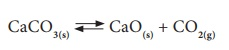Home | | Science 10th Std | State of Equilibrium

# State of Equilibrium

Chemical Equilibrium: It is state of a reversible chemical reaction in which no change in the amount of the reactants and products takes place. At equilibrium, Rate of forward reaction = Rate of backward reaction

STATE OF EQUILIBRIUM

In a reversible reaction, both forward and backward reactions take place simultaneously. When the rate of the forward reaction becomes equal to the rate of backward reaction, then no more product is formed. This stage of the reaction is called ‘equilibrium state’ . After this stage, no net change in the reaction can occur and hence in the amount of the reactants and products. Since this equilibrium is attained in a chemical reaction, it is called ‘Chemical Equilibrium’. Chemical Equilibrium: It is state of a reversible chemical reaction in which no change in the amount of the reactants and products takes place. At equilibrium,

Rate of forward reaction = Rate of backward reaction

## Explanation:

Initially the rate of the forward reaction is greater than the rate of the backward reaction. However, during the course of reaction, the concentration of the reactants decreases and the concentration of the products increases. Since the rate of a reaction is directly proportional to the concentration, the rate of the forward reaction decreases with time, whereas the rate of the backward reaction increases.

At a certain stage, both the rates become equal. From this point onwards, there will be no change in the concentrations of both the reactants and the products with time. This state is called as equilibrium state.

Let us consider the decomposition of calcium carbonate into lime and carbon dioxide. It is a reversible reaction. The speed of each reaction can be determined by how quickly the reactant disappears. If the reaction is carried out in a closed vessel, it reaches a chemical equilibrium. At this stage,The rate of decomposition of CaCO3 = The rate of combination of CaO and CO2

Not only chemical changes, physical changes also may attain equilibrium. When water kept in a closed vessel evaporates, it forms water vapour. No water vapour escapes out of the container as the process takes place in a closed vessel. So, it builds up the vapour pressure in the container. At one time, the water vapour condenses back into liquid water and when the rate of this condensation becomes equal to that of vapourisation, the process attains equilibrium.

At this stage, the volume of the liquid and gaseous phases remain constant. Since it is a physical change, the equilibrium attained is called ‘Physical Equilibrium’. Physical equilibrium is a state of a physical change at which the volume of all the phases remain unchanged.## Characteristics of equilibrium

·                 In a chemical equilibrium, the rates of the forward and backward reactions are equal.

·                 The observable properties such as pressure, concentration, colour, density, viscosity etc., of the system remain unchanged with time.

·                 The chemical equilibrium is a dynamic equilibrium, because both the forward and backward reactions continue to occur even though it appears static externally.

·                 In physical equilibrium, the volume of all the phases remain constant.

Study Material, Lecturing Notes, Assignment, Reference, Wiki description explanation, brief detail
10th Science : Chapter 10 : Types of Chemical Reactions : State of Equilibrium |